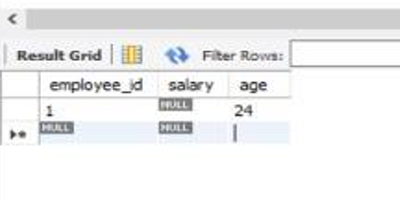# What is the difference between null=True and blank=True in Django?

Django | null=True vs blank=True: Learn about the differences between null=True and blank=True.
Submitted by Apurva Mathur, on May 15, 2022

Let us first distinguish between the Null value and the Blank value in DBMS.

Null Value: In terms of database management system, a null value is something that doesn't exist. A field with null value is a field with no value. Basically it is different from "0" or any real value. Usually, the null value tells us that value exists but it is not known or undefined for that row.

Example:

For example, if the salary of a particular employee is not available in the salary column of the Employee table then it will be represented as null but not as zero.In the above picture, under the salary column, no value is inputted so it is showing NULL.

Blank Value: Also known as an empty value, basically a Blank value is like an empty string of text or a string that only contains white space tabs, or we can also say that a string value contains 0 characters.

Example:

For example, on a web application when we fail to complete a field of the enrollment form like sometimes we leave a column of phone number or age, then, in this case, blank values will be submitted.

In the picture given below phone, number column is left blank which means an empty string is there as an input.The same concepts apply in Django also,

In Django blank="true" means, the field allows the empty value like (white space tabs, empty string). This means if in any model this condition is applied that particular field will take an empty string as an input.

Whereas the case will be reversed if blank="false" is applied, as in this case, the model field will prevent empty values from being inputted.

Example:

```from django.db import models

class Book(models.Model):
name=models.CharField(max_length=20)
author = models.CharField(max_length=20)

def __str__(self):
return self.name
```

The above example will give a better understanding of the blank=true field. Here, the about field is given the parameter blank=true which means that the user doesn't have to put any legitimate value to fill this field.

On the other hand, null tells our database that the field can be left empty. If the condition is null="true" is encountered then in this case it specifies that a particular field in a model can be left empty.

Whereas in another case where null="false" is applied in a model field then the user has to input some value else the model will show an error (usually called Integrity error).

Example:

```from django.db import models

class Book(models.Model):
name=models.CharField(max_length=20)
author = models.CharField(max_length=20)
date = models.DateField(null=True,blank=True)

def __str__(self):
return self.name
```

In this example, the date field has parameters null=true which indicates that the default value of this field is set to null.

Important Note:

If we are making a field that can have a garbage value, then in a case like that it is essential to make null=true, so it does not return any runtime error while running the program.

In the end, we can finally conclude that null=true blank=true means that the field is optional in every situation. If no value is inputted by the user then in such a case no error is going to raise.